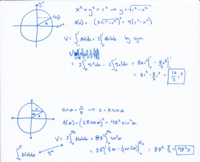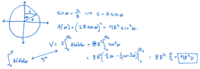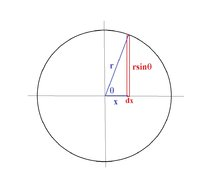# stewart, 8th ed, problem 54

#### EJE12345

##### New member
Stewart, Early Transcendentals, 6.2, problem 54: find volume of solid - base S is a circular disc with radius R. Parallel cross-sections perpendicular to base are squares. I did it 2 ways, and got different answers. First one goes along with the chapter examples, and I think is fine. Second one (integrating with respect to angle theta) seems like it should work, but different answer. Where do I go wrong? thanks

#### Attachments

• 203.1 KB Views: 4

#### EJE12345

##### New member
need help with problem from Stewart's Early Transcendental's, 8th ed, 6.2.54. find volume of solid: base is disk with radius r, parallel cross-sections perpendicular to base squares. i have two solutions: first follows along with the ideas in the examples of the section, finding area of square as a function of point x on the circle, then integrating over 0 to the radius. the second solution i think it wrong but can't tell why - looking at the the area of the square as a function of the angle theta defined in the image, then integrating from 0 to pi/2. where am i going wrong? thanks#### nasi112

##### Junior Member
Do you have the correct answer?

Is the answer in the book $$\displaystyle \frac{16}{3}r^3$$?

#### EJE12345

##### New member
no book answer, even number problem. im pretty sure it is (16/3)R^3; i want to know why the second method is incorrrect, and how to make it correct

#### nasi112

##### Junior Member
First, you have to be sure your first method is correct, then we can go and check the second method.

I think that your first method is wrong. What do you say?

#### EJE12345

##### New member
i am happy to hear why it is incorrect. the solid created is unusual, but really just a bunch of square slices put together. the sides of the squares vary with the radius of the circle. there could be a flaw in the set up certainly.

#### nasi112

##### Junior Member
Your final answer is correct by coincidence because we are moving in a circle, but setting the integral is wrong.

if we let $$\displaystyle 2x$$ be one side of the square, then its area is $$\displaystyle 4x^2$$, then we have to take the height as $$\displaystyle dy$$, not $$\displaystyle dx$$, so the integral is

$$\displaystyle V = \int_{-r}^{r} 4x^2 \ dy = 8\int_{0}^{r} r^2 - y^2 \ dy$$

#### EJE12345

##### New member
i see, i got terms mixed up when transposing from a note book. so you agree the first answer is correct.

do you know why the method is incorrect, using trig functions instead?

i was a math major in college 20y ago, im relearning a lot of this just as a hobby, so i dont have a teacher/professor. the answer to my question may be apparent later as i go through the book. thanks for the help.

#### Dr.Peterson

##### Elite Member
Stewart, Early Transcendentals, 6.2, problem 54: find volume of solid - base S is a circular disc with radius R. Parallel cross-sections perpendicular to base are squares. I did it 2 ways, and got different answers. First one goes along with the chapter examples, and I think is fine. Second one (integrating with respect to angle theta) seems like it should work, but different answer. Where do I go wrong? thanksThe element of volume is not given by $$A(\theta)d\theta$$; the thickness of a slice is not $$d\theta$$.

Have you learned anything about integrating in polar coordinates?

thanks a bunch

#### EJE12345

##### New member
not yet... i cant recall the details of change of coordinates (math major 20y ago, now revisiting stuff) - when i get to polar coordinates in the book ill revisit this.
thanks

#### skeeter

##### Elite Member
$$\displaystyle x = r\cos{\theta} \implies dx = -r\sin{\theta} \, d\theta$$

$$\displaystyle dA = (2r\sin{\theta})^2$$

$$\displaystyle V = \int_{\pi}^0 (4r^2\sin^2{\theta})(-r\sin{\theta}) \, d\theta$$

note ... rotating $$\displaystyle \theta \text{ from } \pi \to 0 \implies dx > 0$$

$$\displaystyle V = 4r^3 \int_{\pi}^0 (1-\cos^2{\theta})(-\sin{\theta}) \, d\theta$$

$$\displaystyle u = \cos{\theta} \implies du = -\sin{\theta} \, d\theta$$

$$\displaystyle V = 4r^3 \int_{-1}^1 (1-u^2) \, du = 4r^3 \bigg[u - \frac{u^3}{3}\bigg]_{-1}^1 = 4r^3\bigg[\frac{2}{3} - \left(-\frac{2}{3}\right)\bigg] = \frac{16r^3}{3}$$#### nasi112

##### Junior Member
i see, i got terms mixed up when transposing from a note book. so you agree the first answer is correct.

do you know why the method is incorrect, using trig functions instead?

i was a math major in college 20y ago, im relearning a lot of this just as a hobby, so i dont have a teacher/professor. the answer to my question may be apparent later as i go through the book. thanks for the help.
First, I apologize to you because I was wrong. Your set up for the first integral is correct. I have just realized that you have used $$\displaystyle 2y$$ for one side of the square instead of $$\displaystyle 2x$$ which is also correct.

To do this in trigonometry is very easy. You can follow skeeter way, or you can continue with my integral.

Last time, we had this integral,

$$\displaystyle V = \int_{-r}^{r} 4x^2 \ dy = 8\int_{0}^{r} r^2 - y^2 \ dy$$

Change the variables to polar coordinate.

$$\displaystyle y = r\sin\theta$$
$$\displaystyle dy = r\cos\theta \ d\theta$$

The integral will become,

$$\displaystyle V = 8\int_{0}^{\pi/2} (r^2 - r^2\sin^2 \theta) (r \cos \theta) \ d\theta = \frac{16r^3}{3}$$

Can you figure out why we chose the limit of integration as $$\displaystyle 0\leq \theta \leq \frac{\pi}{2}$$?

#### EJE12345

##### New member
thanks guys for doing that. i do recall change of variables from an analysis course 20y ago... looks like this is a few chapters ahead in the book